# 7. Discretized Non-negative Tucker Decomposition (dNTD)

Laboratory for Bioinformatics Research, RIKEN Center for Biosystems Dynamics Research

# Introduction

In this vignette, we consider approximating a non-negative tensor as a product of binary or non-negative low-rank matrices (a.k.a., factor matrices).

Test data is available from toyModel.

library("dcTensor")
X <- dcTensor::toyModel("dNTF")

You will see that there are four blocks in the data tensor as follows.

suppressMessages(library("nnTensor"))
plotTensor3D(X)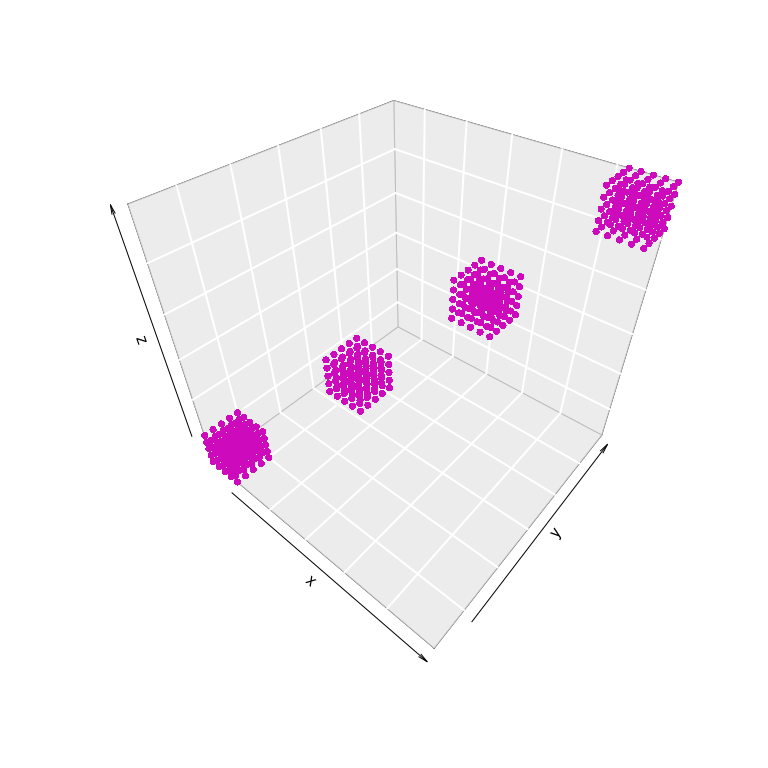# Binary Tensor Factorization (BTF)

Here, we introduce a non-negative tensor decomposition method, non-negative Tucker decomposition (NTD (Kim 2007; CICHOCK 2009)). The difference with the NTF is that different ranks can be specified for factor matrices such as $$A_1$$ ($$J1 \times N$$), $$A_2$$ ($$J2 \times M$$), and $$A_3$$ ($$J3 \times L$$) and that the core tensor can have non-negative values in the non-diagonal elements. This degree of freedom will show that NTD fits the second set of data well. For the details, see NTD function of nnTensor package.

## Basic Usage

In BTF by NTD, rank parameters are needed to be set in advance. Other settings such as the number of iterations (num.iter) or factorization algorithm (algorithm) are also available. For the details of arguments of dNTD, see ?dNTD. After the calculation, various objects are returned by dNTD. BTF is achieved by specifying the binary regularization parameter as a large value like the below:

set.seed(123456)
out_dNTD <- dNTD(X, Bin_A=c(1e+6, 1e+6, 1e+6), thr=1e-20, rank=c(4,4,4))
str(out_dNTD, 2)
## List of 6
##  $S :Formal class 'Tensor' [package "rTensor"] with 3 slots ##$ A            :List of 3
##   ..$A1: num [1:4, 1:30] 0 0 0.000353 0 0 ... ## ..$ A2: num [1:4, 1:30] 0 1 0 0 0 ...
##   ..$A3: num [1:4, 1:30] 0 1 0 0 0 ... ##$ RecError     : Named num [1:101] 1.00e-19 3.10e-14 3.14e-14 3.10e-14 3.07e-14 ...
##   ..- attr(*, "names")= chr [1:101] "offset" "1" "2" "3" ...
##  $TrainRecError: Named num [1:101] 1.00e-19 3.10e-14 3.14e-14 3.10e-14 3.07e-14 ... ## ..- attr(*, "names")= chr [1:101] "offset" "1" "2" "3" ... ##$ TestRecError : Named num [1:101] 1e-19 0e+00 0e+00 0e+00 0e+00 ...
##   ..- attr(*, "names")= chr [1:101] "offset" "1" "2" "3" ...
##  $RelChange : Named num [1:101] 1.00e-19 8.96e+16 1.42e-02 1.27e-02 8.93e-03 ... ## ..- attr(*, "names")= chr [1:101] "offset" "1" "2" "3" ... After the calculation, various objects are returned by dNTD. The reconstruction error (RecError) and relative error (RelChange, the amount of change from the reconstruction error in the previous step) can be used to diagnose whether the calculation is converged or not. layout(t(1:2)) plot(log10(out_dNTD$RecError[-1]), type="b", main="Reconstruction Error")
plot(log10(out_dNTD$RelChange[-1]), type="b", main="Relative Change")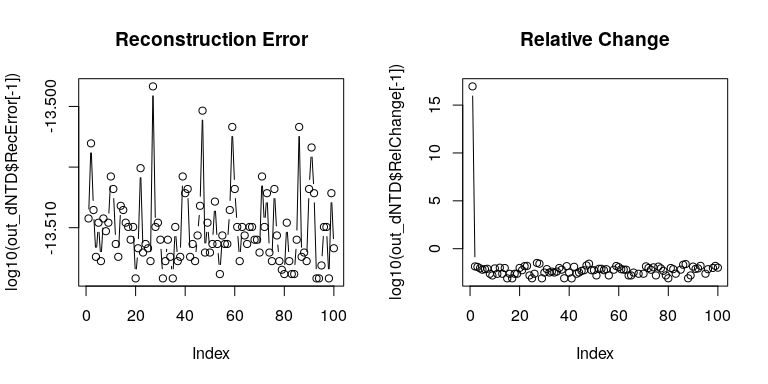The product of core tensor $$S$$ and factor matrices $$A_{k}$$ shows whether the original data is well-recovered by dNTD. recX <- recTensor(out_dNTD$S, out_dNTD$A) layout(t(1:2)) plotTensor3D(X) plotTensor3D(recX, thr=0)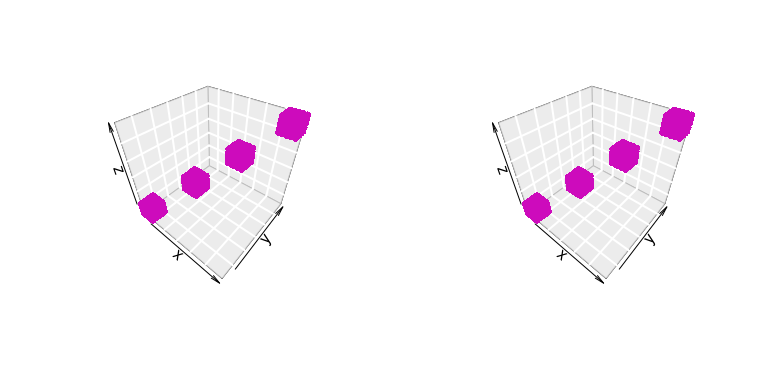The histograms of $$A_{k}$$s show that $$A_{k}$$ looks binary. layout(t(1:3)) hist(out_dNTD$A[], main="A1", breaks=100)
hist(out_dNTD$A[], main="A2", breaks=100) hist(out_dNTD$A[], main="A3", breaks=100)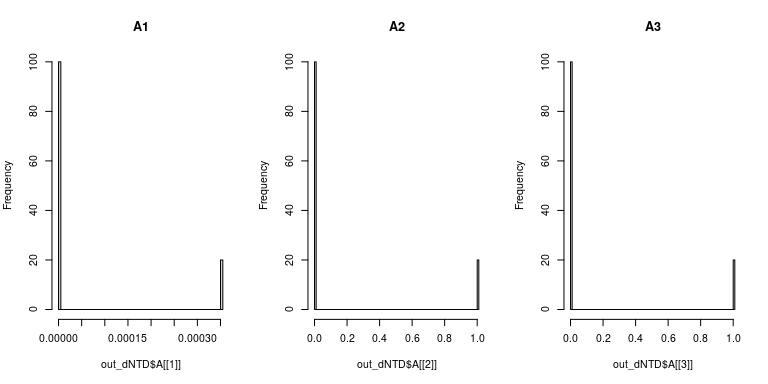# Semi-Binary Tensor Factorization (SBTF)

Here, we define this formalization as semi-binary tensor factorization (SBTF). SBTF can capture discrete patterns from non-negative matrices.

To demonstrate SBMF, next we use a non-negative tensor from the nnTensor package. You will see that there are four blocks in the data tensor as follows.

X2 <- nnTensor::toyModel("CP")
plotTensor3D(X2)## Basic Usage

In SBTF by NTD, rank parameters are needed to be set in advance. Other settings such as the number of iterations (num.iter) or factorization algorithm (algorithm) are also available. For the details of arguments of dNTF, see ?dNTF. After the calculation, various objects are returned by dNTF. SBTF is achieved by specifying the binary regularization parameter as a large value like the below:

set.seed(123456)
out_dNTD2 <- dNTD(X2, Bin_A=c(1e-10, 1e-10, 1e+5), rank=c(4,4,4))
str(out_dNTD2, 2)
## List of 6
##  $S :Formal class 'Tensor' [package "rTensor"] with 3 slots ##$ A            :List of 3
##   ..$A1: num [1:4, 1:30] 1.29e-08 1.22e-08 6.94e-06 1.04e-08 2.54e-08 ... ## ..$ A2: num [1:4, 1:30] 7.89e-02 1.05e+01 1.73e-16 3.85e-02 3.94e-02 ...
##   ..$A3: num [1:4, 1:30] 0.001979 1.019649 0.001033 0.002067 0.000236 ... ##$ RecError     : Named num [1:101] 1.00e-09 2.92e+02 2.92e+02 2.92e+02 2.92e+02 ...
##   ..- attr(*, "names")= chr [1:101] "offset" "1" "2" "3" ...
##  $TrainRecError: Named num [1:101] 1.00e-09 2.92e+02 2.92e+02 2.92e+02 2.92e+02 ... ## ..- attr(*, "names")= chr [1:101] "offset" "1" "2" "3" ... ##$ TestRecError : Named num [1:101] 1e-09 0e+00 0e+00 0e+00 0e+00 0e+00 0e+00 0e+00 0e+00 0e+00 ...
##   ..- attr(*, "names")= chr [1:101] "offset" "1" "2" "3" ...
##  $RelChange : Named num [1:101] 1.00e-09 1.12e+07 1.09e-03 9.36e-04 7.81e-04 ... ## ..- attr(*, "names")= chr [1:101] "offset" "1" "2" "3" ... RecError and RelChange can be used to diagnose whether the calculation is converged or not. layout(t(1:2)) plot(log10(out_dNTD2$RecError[-1]), type="b", main="Reconstruction Error")
plot(log10(out_dNTD2$RelChange[-1]), type="b", main="Relative Change")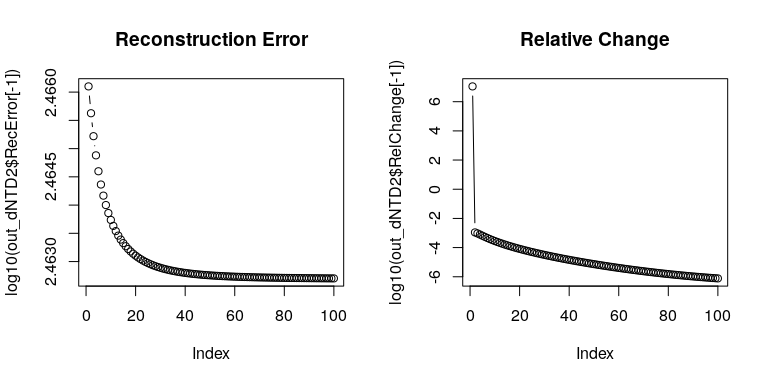The product of core tensor $$S$$ and factor matrices $$A_{k}$$ shows whether the original data is well-recovered by dNTD. recX <- recTensor(out_dNTD2$S, out_dNTD2$A) layout(t(1:2)) plotTensor3D(X2) plotTensor3D(recX, thr=0)The histograms of $$A_{k}$$s show that $$A_{k}$$ looks binary. layout(t(1:3)) hist(out_dNTD2$A[], main="A1", breaks=100)
hist(out_dNTD2$A[], main="A2", breaks=100) hist(out_dNTD2$A[], main="A3", breaks=100)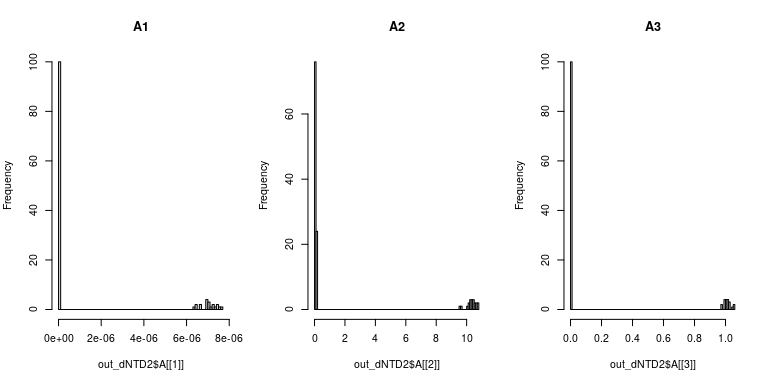# Session Information

## R version 4.3.0 (2023-04-21)
## Platform: x86_64-pc-linux-gnu (64-bit)
## Running under: Ubuntu 22.04.2 LTS
##
## Matrix products: default
## LAPACK: /usr/lib/x86_64-linux-gnu/openblas-pthread/libopenblasp-r0.3.20.so;  LAPACK version 3.10.0
##
## locale:
##   LC_CTYPE=en_US.UTF-8       LC_NUMERIC=C
##   LC_TIME=en_US.UTF-8        LC_COLLATE=C
##   LC_MONETARY=en_US.UTF-8    LC_MESSAGES=en_US.UTF-8
##   LC_PAPER=en_US.UTF-8       LC_NAME=C
##  LC_MEASUREMENT=en_US.UTF-8 LC_IDENTIFICATION=C
##
## time zone: Etc/UTC
## tzcode source: system (glibc)
##
## attached base packages:
##  stats     graphics  grDevices utils     datasets  methods   base
##
## other attached packages:
##  nnTensor_1.1.13   fields_14.1       viridis_0.6.3     viridisLite_0.4.2
##  spam_2.9-1        dcTensor_1.2.0
##
## loaded via a namespace (and not attached):
##   gtable_0.3.3       jsonlite_1.8.5     highr_0.10         dplyr_1.1.2
##   compiler_4.3.0     maps_3.4.1         Rcpp_1.0.10        tagcloud_0.6
##   plot3D_1.4         tidyselect_1.2.0   gridExtra_2.3      jquerylib_0.1.4
##  scales_1.2.1       yaml_2.3.7         fastmap_1.1.1      ggplot2_3.4.2
##  R6_2.5.1           tcltk_4.3.0        generics_0.1.3     knitr_1.43
##  MASS_7.3-60        misc3d_0.9-1       dotCall64_1.0-2    tibble_3.2.1
##  munsell_0.5.0      RColorBrewer_1.1-3 bslib_0.5.0        pillar_1.9.0
##  rlang_1.1.1        utf8_1.2.3         cachem_1.0.8       xfun_0.39
##  sass_0.4.6         cli_3.6.1          magrittr_2.0.3     digest_0.6.31
##  grid_4.3.0         rTensor_1.4.8      lifecycle_1.0.3    vctrs_0.6.2
##  evaluate_0.21      glue_1.6.2         fansi_1.0.4        colorspace_2.1-0
##  rmarkdown_2.22     tools_4.3.0        pkgconfig_2.0.3    htmltools_0.5.5